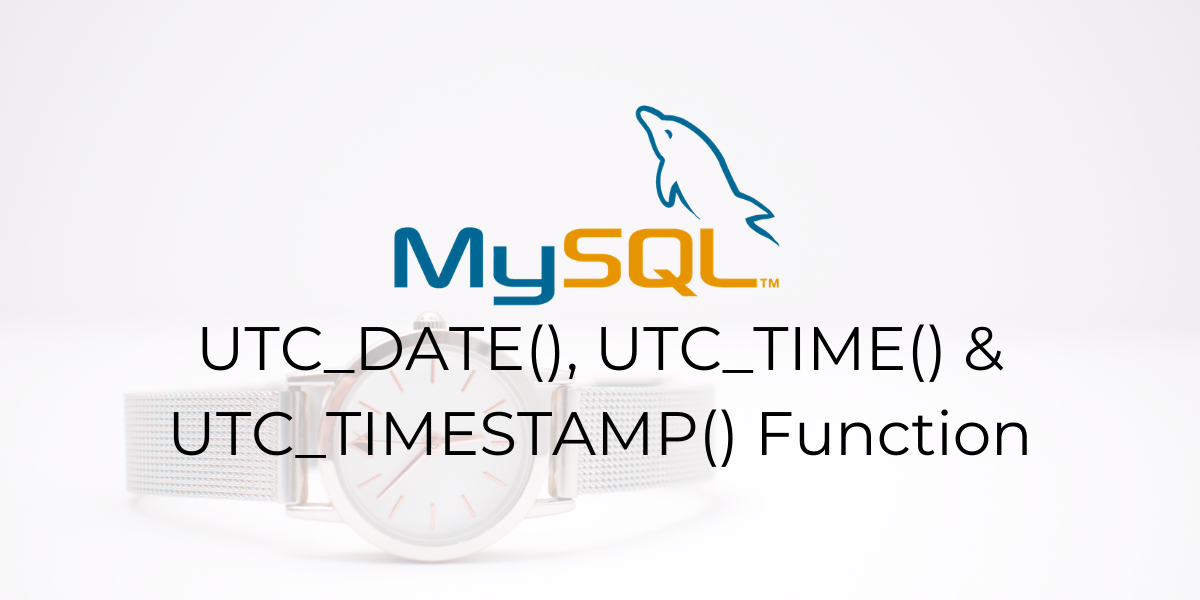# MySQL UTC_TIME(), UTC_DATE() and UTC_TIMESTAMP() functionsIn this tutorial, we will study the `MySQL UTC_TIME(), UTC_DATE() and UTC_TIMESTAMP()` functions. Coordinated Universal Time or UTC is the primary time standard by which the world regulates clocks and time.

It is within about 1 second of mean solar time at 0° longitude, and is not adjusted for daylight saving time.

It is effectively a successor to Greenwich Mean Time (GMT). Suppose you want to find the UTC time in MySQL. This is where you use the `UTC_TIME()` function.

Furthermore, we also have `UTC_DATE()` and `UTC_TIMESTAMP()` functions. Let us take a look at this.

• The `UTC_TIME()` function is used to return the current UTC time.
• The `UTC_DATE()` function is used to return the current UTC date.
• The `UTC_TIMESTAMP()` function is used to return the current UTC datetime value.

Let us take a look at the syntax and example of each of them.

## Syntax of MySQL UTC_TIME()

```UTC_TIME([fsp])
```

Or

```UTC_TIME
```

Where,

‘fsp’ is an optional parameter that states the fractional seconds precision for the time value to be returned.

## Syntax of MySQL UTC_DATE()

```UTC_DATE()
```

Or

```UTC_DATE
```

## Syntax of MySQL UTC_TIMESTAMP()

```UTC_TIMESTAMP([fsp])
```

Or

```UTC_TIMESTAMP
```

Where,

‘fsp’ is an optional parameter that states the fractional seconds precision for the datetime value to be returned.

## Examples of MySQL UTC_TIME()

Let us start with the `MySQL UTC_TIME()` function. Here is how we can display the current UTC time using the `SELECT` statement.

```SELECT UTC_TIME();
```

And the output is –

We can also do it without the parentheses as follows –

```SELECT UTC_TIME;
```

And the output is –

### Displaying Fractional Seconds Precision of the current UTC time.

We can display the UTC time value upto 6 fractional seconds precision. In this case, we need to specify the ‘fsp’ parameter. The ‘fsp’ parameter can have a value from 1 to 6. Let us see an example of this.

```SELECT UTC_TIME(6);
```

And the output is –

### MySQL UTC_TIME() in Numerical Context

In the above examples, we got the time in the HH:MM:SS format. This is because the time value was returned as a string. To return the time value in a numerical context, we add a “+ 0” to it. Here is an example of this.

```SELECT UTC_TIME() + 0;
```

And the output is –

## Examples of MySQL UTC_DATE()

Next, let us move on with the `UTC_DATE()` function. Here are the two ways you can display the current UTC date using the `SELECT` statement. The queries are –

```SELECT UTC_DATE;
SELECT UTC_DATE();
```

And the output is –

### UTC Date in Numerical Context

In the above example, we got the date in the ‘YYYY-MM-DD’ format. This is because the date value was returned as a string. To return the date value in a numerical context, we add a “+ 0” to it. In the below example we add “+ 5” to the `UTC_DATE()` function. This returns the numerical value for the date 5 days after the current UTC date.

```SELECT UTC_DATE() + 5;
```

And the output is –

## Examples of MySQL UTC_TIMESTAMP()

Finally, let us see examples of the `UTC_TIMESTAMP()` function. Here are the two ways you can display the current UTC datetime value using the `SELECT` statement. The queries are –

```SELECT UTC_TIMESTAMP;
SELECT UTC_TIMESTAMP();
```

And the output is –

### Displaying Fractional Seconds Precision of the current UTC datetime value.

We can display the UTC datetime value upto 6 fractional seconds precision. In this case, we need to specify the ‘fsp’ parameter. The ‘fsp’ parameter can have a value from 1 to 6. Let us see an example of this.

```SELECT UTC_TIMESTAMP(6);
```

And the output is –

### UTC datetime value in Numerical Context

In the above examples, we got the datetime in the ‘YYYY-MM-DD HH:MM:SS’ format. This is because the datetime value was returned as a string. To return the datetime value in a numerical context, we add a “+ 0” to it. Here is an example of this.

```SELECT UTC_TIMESTAMP() + 0, UTC_TIMESTAMP() + 5;
```

And the output is –

## Conclusion

So that was a roundup on how we display the UTC time, date and timestamp using MySQL. I hope you liked this article. Do check out the below references.